# OECD Gender wage pay gap data analysis 6 - Multiple Linear Regression with RUnsplashMatteo Vellaが撮影した写真

This post is following of the above post.
In the above post, I did simple linear regression analysis. This time, I will for multiple linear regression (MLR).

I make a new variable, factor of TIME.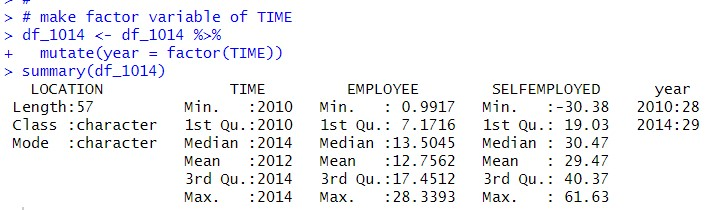I made "year" which is factor type variable representing "TIME". I see 2010 has 28 observations and 2014 has 29 observations.

Let's do MLR (Multiple Linear Regression).
First, I make an interaction model.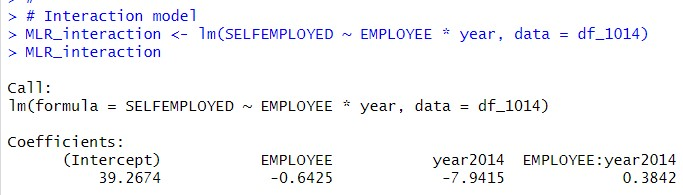Interaction model has two intercepts and two slopes.

If year == 2014,

SELFEMPLOYED = 39.2674 - 7.9415 + (-0.6425 + 0.3842) * EMPLOYEE + u

= 31.3529 - 0.2583 * EMPLOYEE + u

If year == 2010,

SELFEMPLOYED = 39.2674 - 0.6425 * EMPLOYEE + u

Let's make a visualization.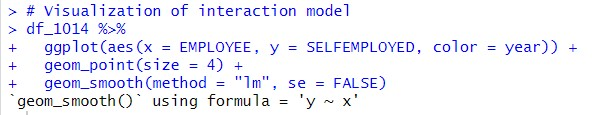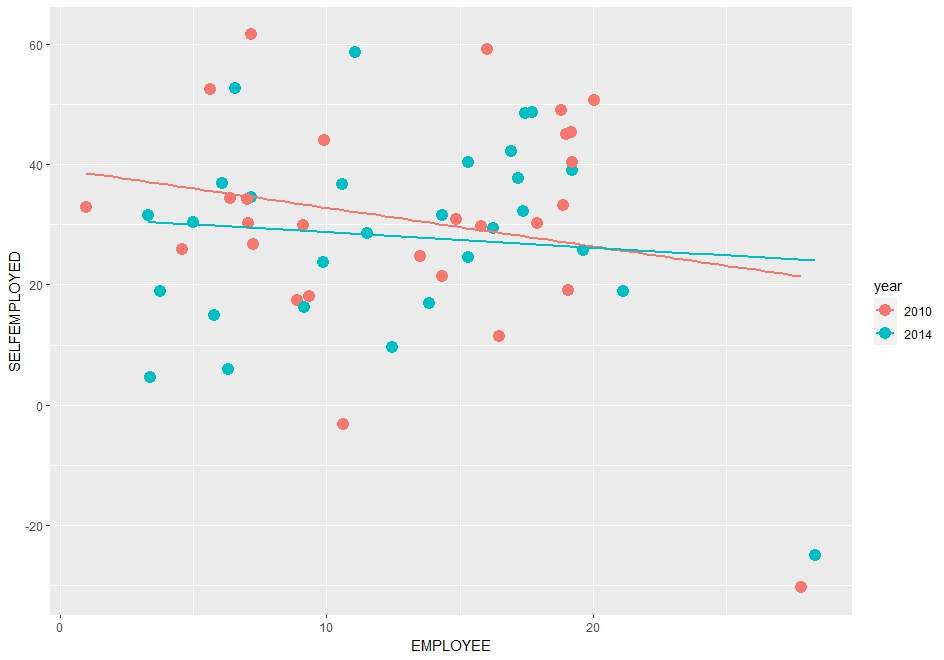Next, I make a parallel slope model.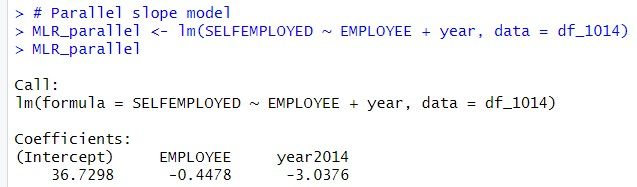If year == 2014, parallel slope model is

SELFEMPLOYEE = 36.7298 - 3.0376 - 0.4478 * EMPLOYEE

= 33.6922 - 0.4478 * EMPLOYEE

If year == 2010,

SELFEMPLOYEE = 36.7298 - 0.4478 * EMPLOYEE.

Let's make a visualization graph.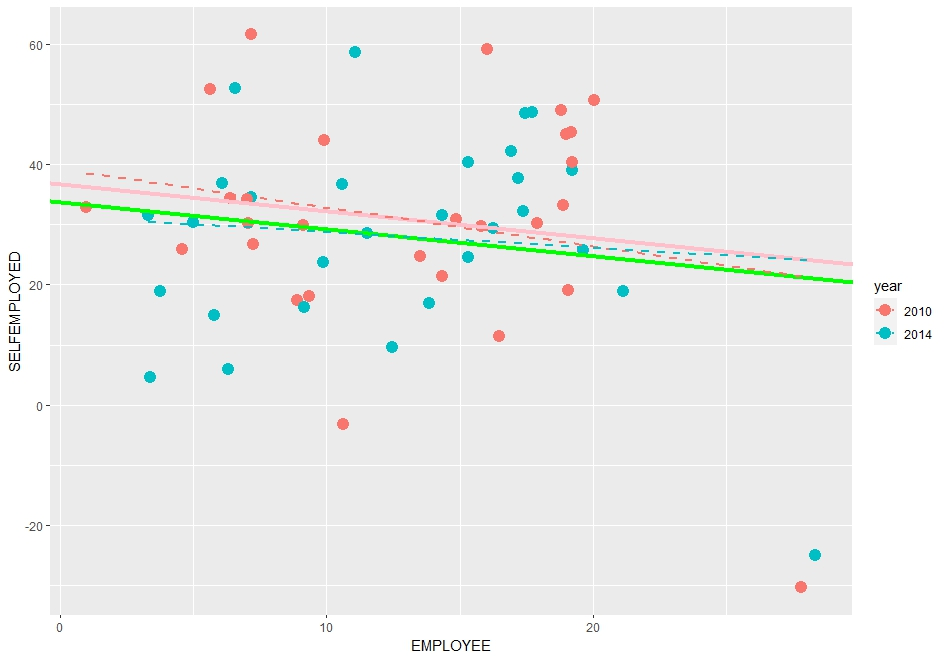solid lines are parallel slope model line, dashed lines are interation model line.

I don't see large difference between parallel slope model and interaction model.

Let's see ragresstion result table with get_regression_table() function of moderndive package.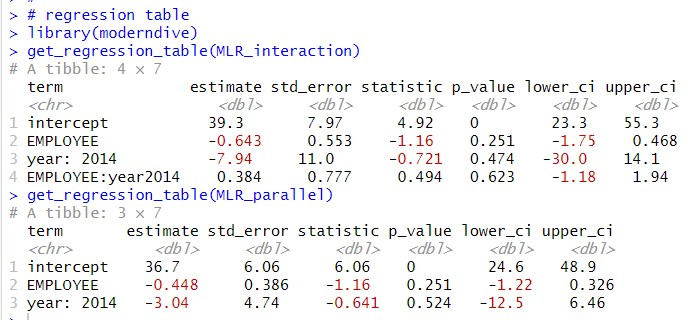Interaction term, EMPLOYEE:year2014 p_value is 0.623 so, it is not significant.

But I found other variables, EMPLOYEE and year: 2014 are also not statistically significant.

So, the both model are not statistically significant.

Let's see regression summary with get_regression_summaries() function.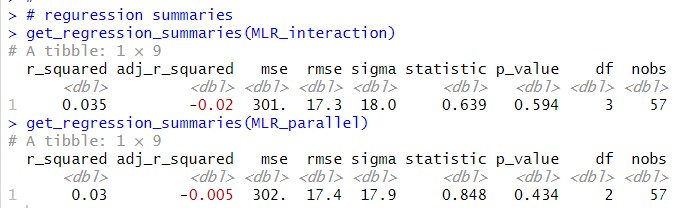p_value of interaction model is 0.594 and one of parallel slope model is 0.434.
So, the both MLR models are not statisticaly significant.

That's it! Thank you!

Next post is

To read from the 1st post,